# RS Aggarwal Solutions for Class 7 Maths Exercise 4B Chapter 4 Rational Numbers

RS Aggarwal Solutions for Class 7 Maths Exercise 4B Chapter 4 Rational Numbers in simple PDF are given here. This exercise of RS Aggarwal Solutions for Class 7 Chapter 4 Rational Numbers contains questions related to representation of rational numbers on the real line and comparison of rational numbers. In the previous class you have learnt how to represent integers on the number line, let us review it by representing the rational numbers on the number line. Students are suggested to try solving the questions from RS Aggarwal Solutions for Class 7 Maths Exercise 4B Chapter 4 Rational Numbers.

## Download the PDF of RS Aggarwal Solutions For Class 7 Maths Chapter 4 Rational Numbers – Exercise 4B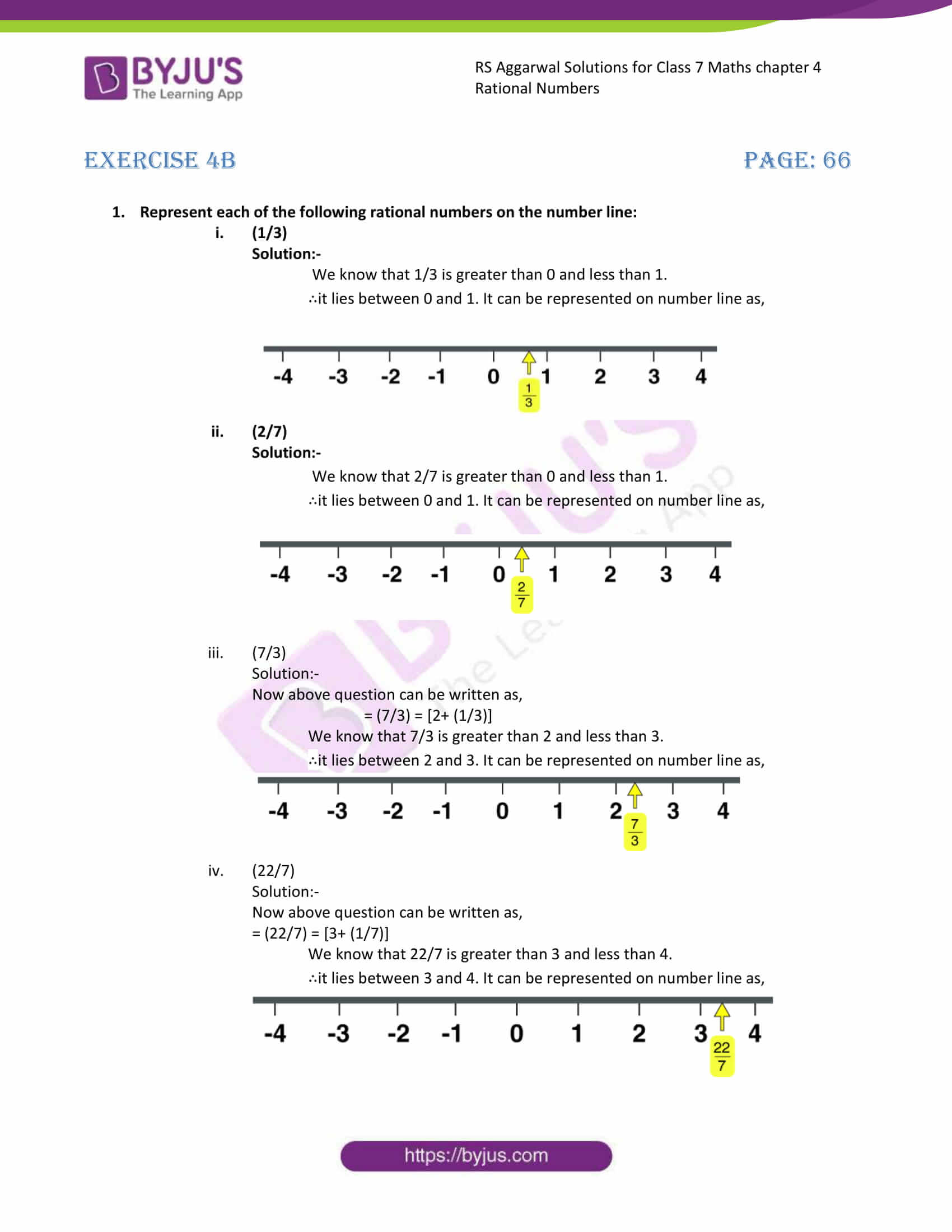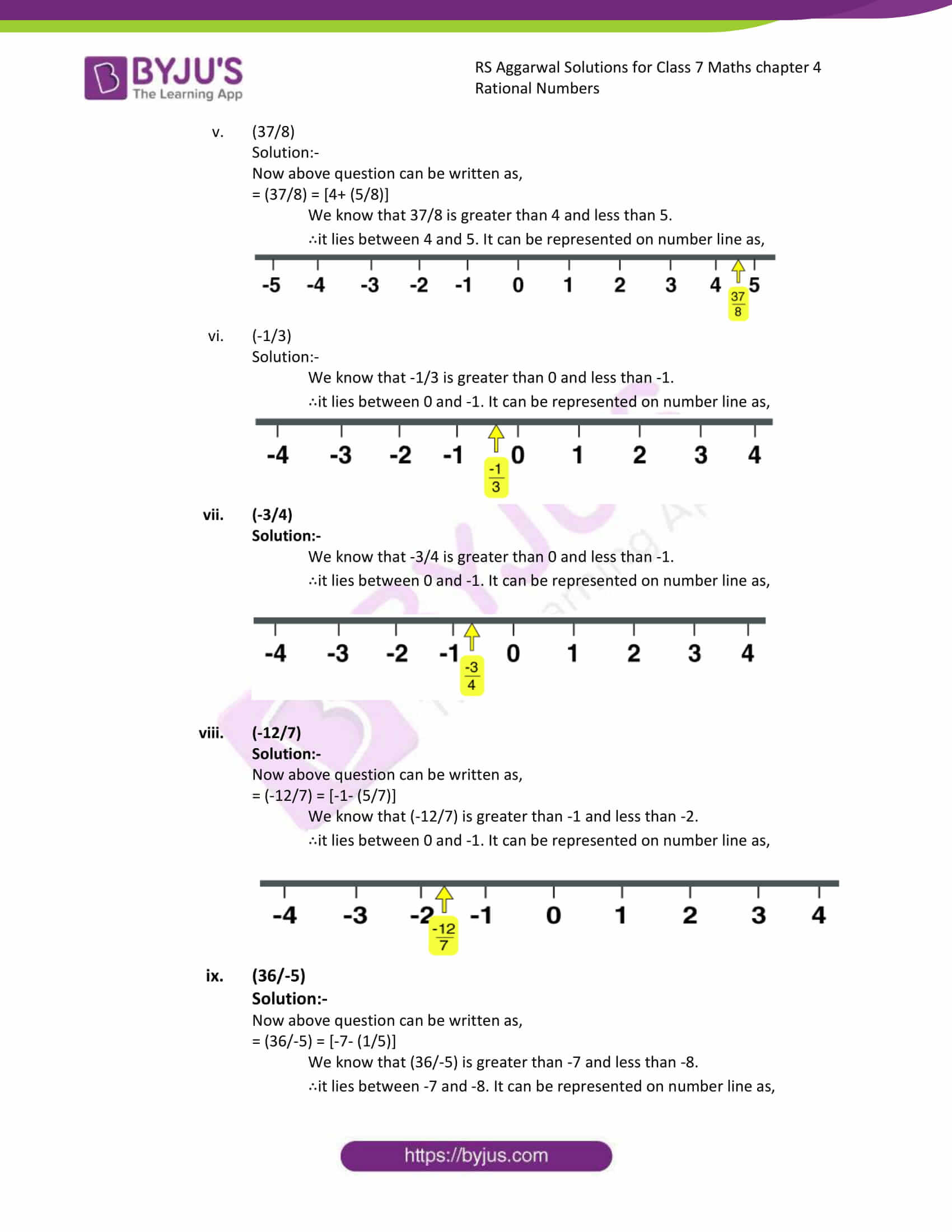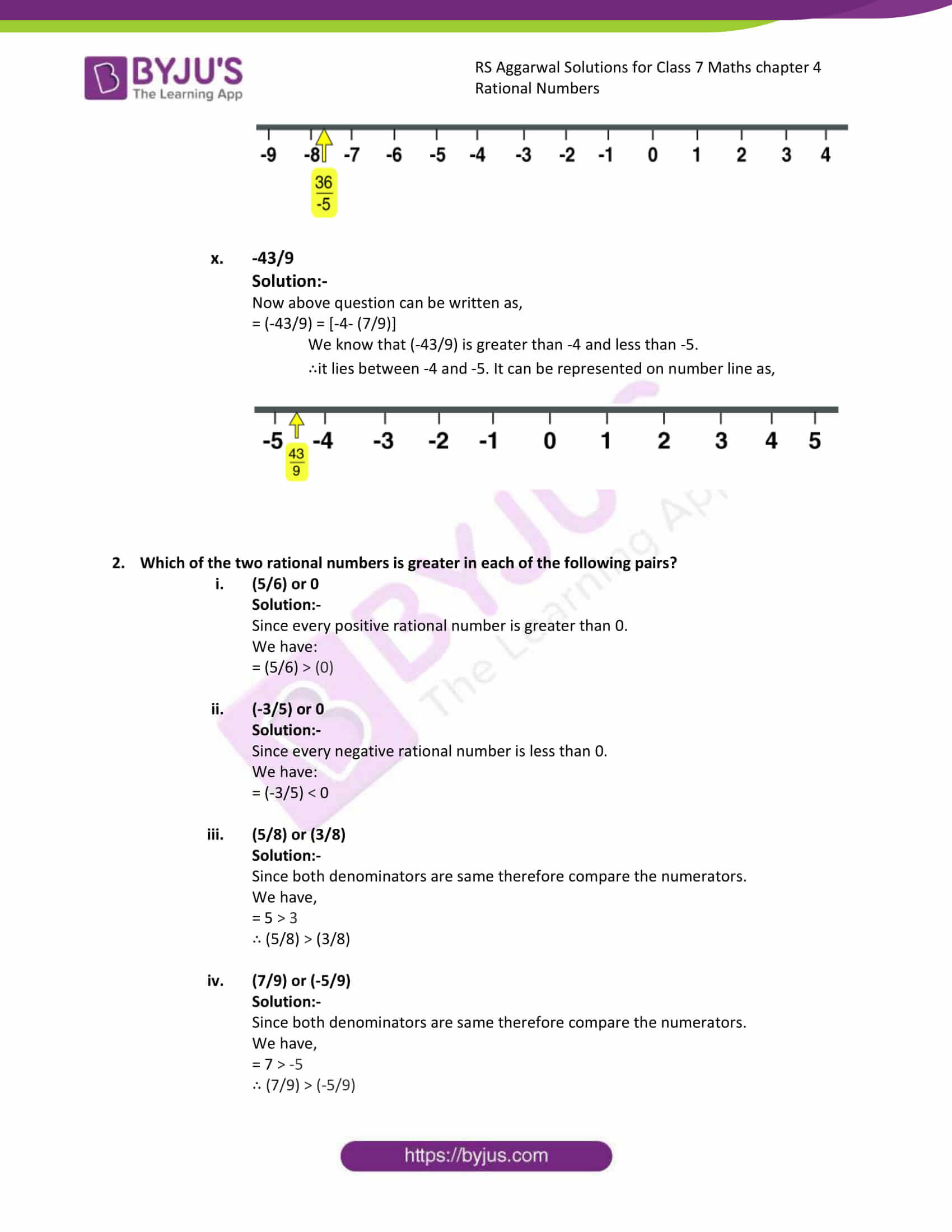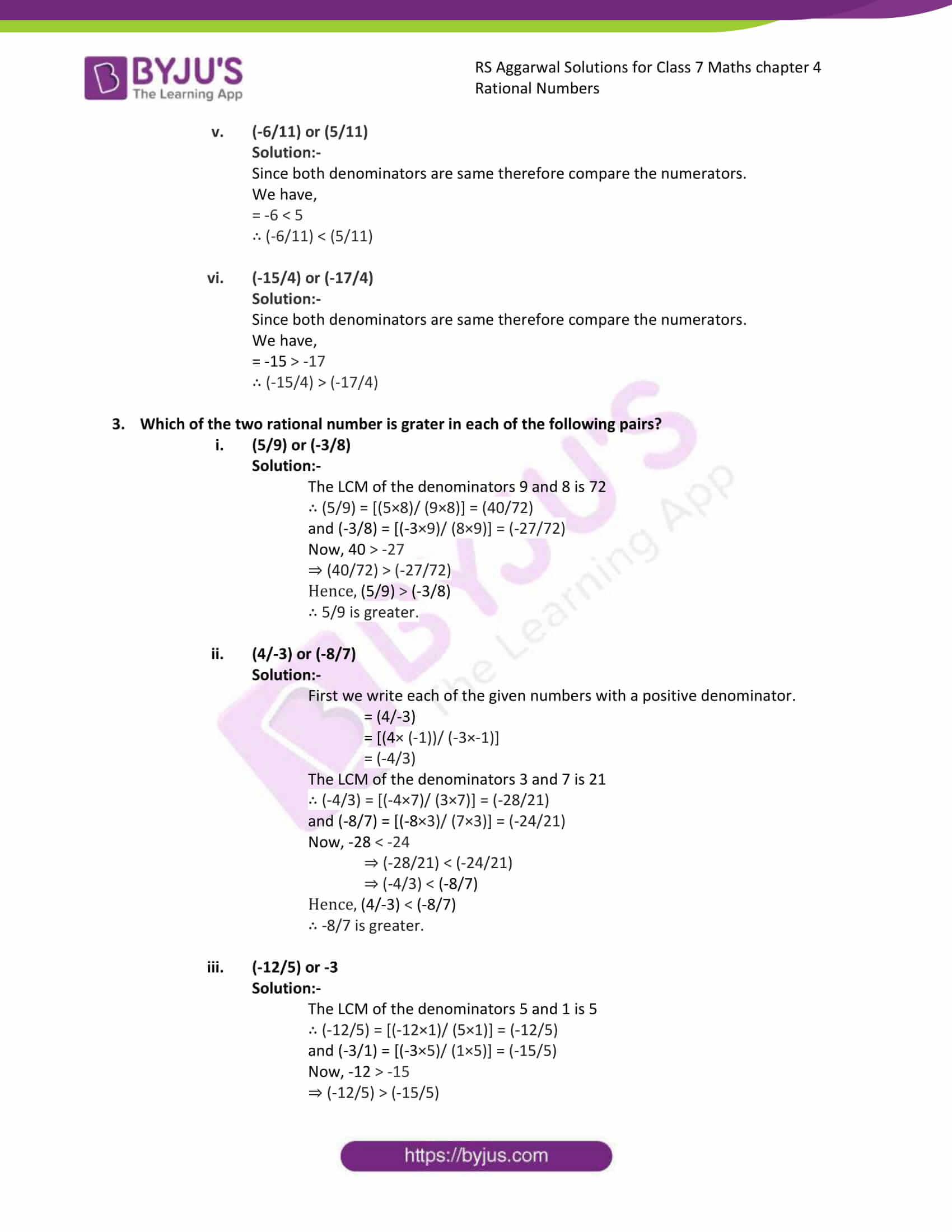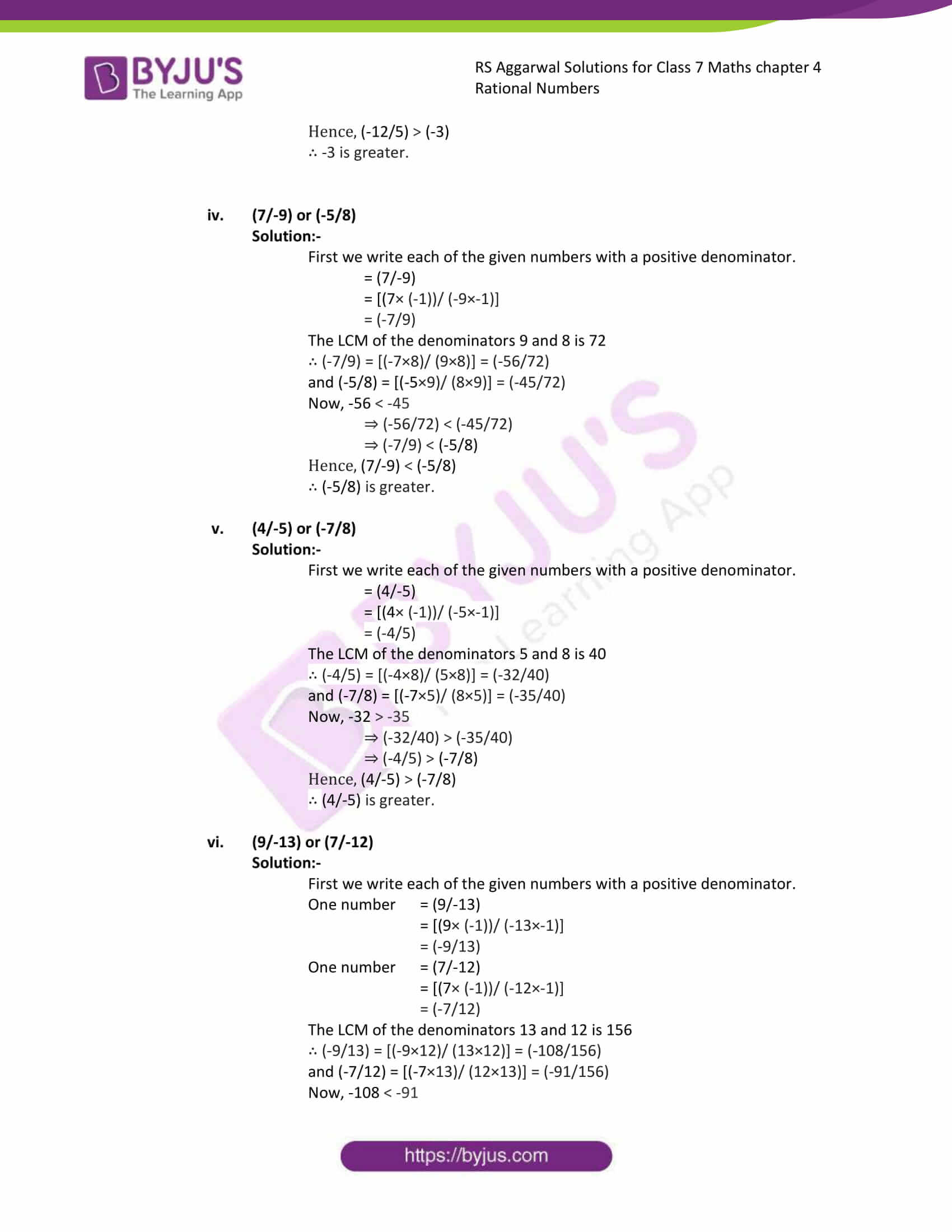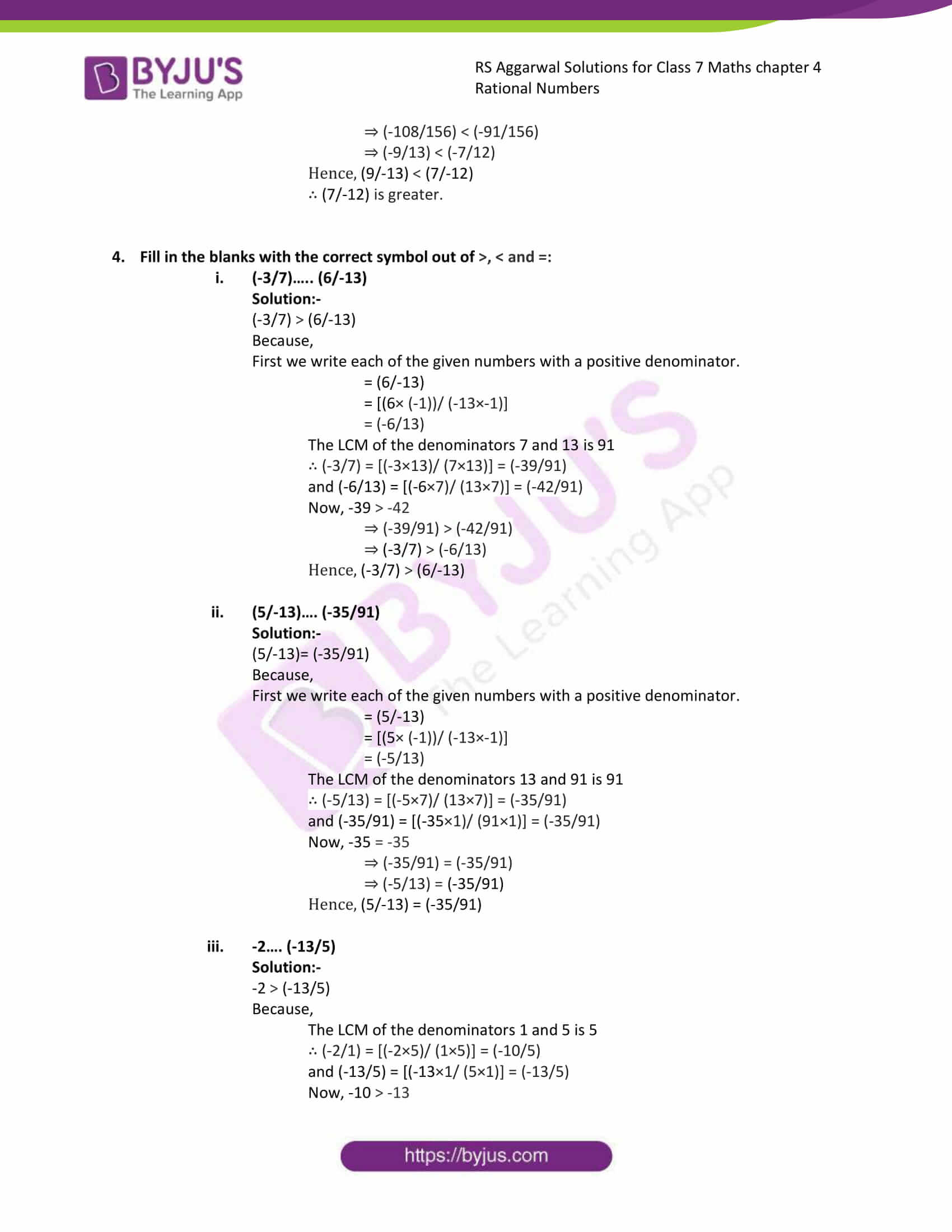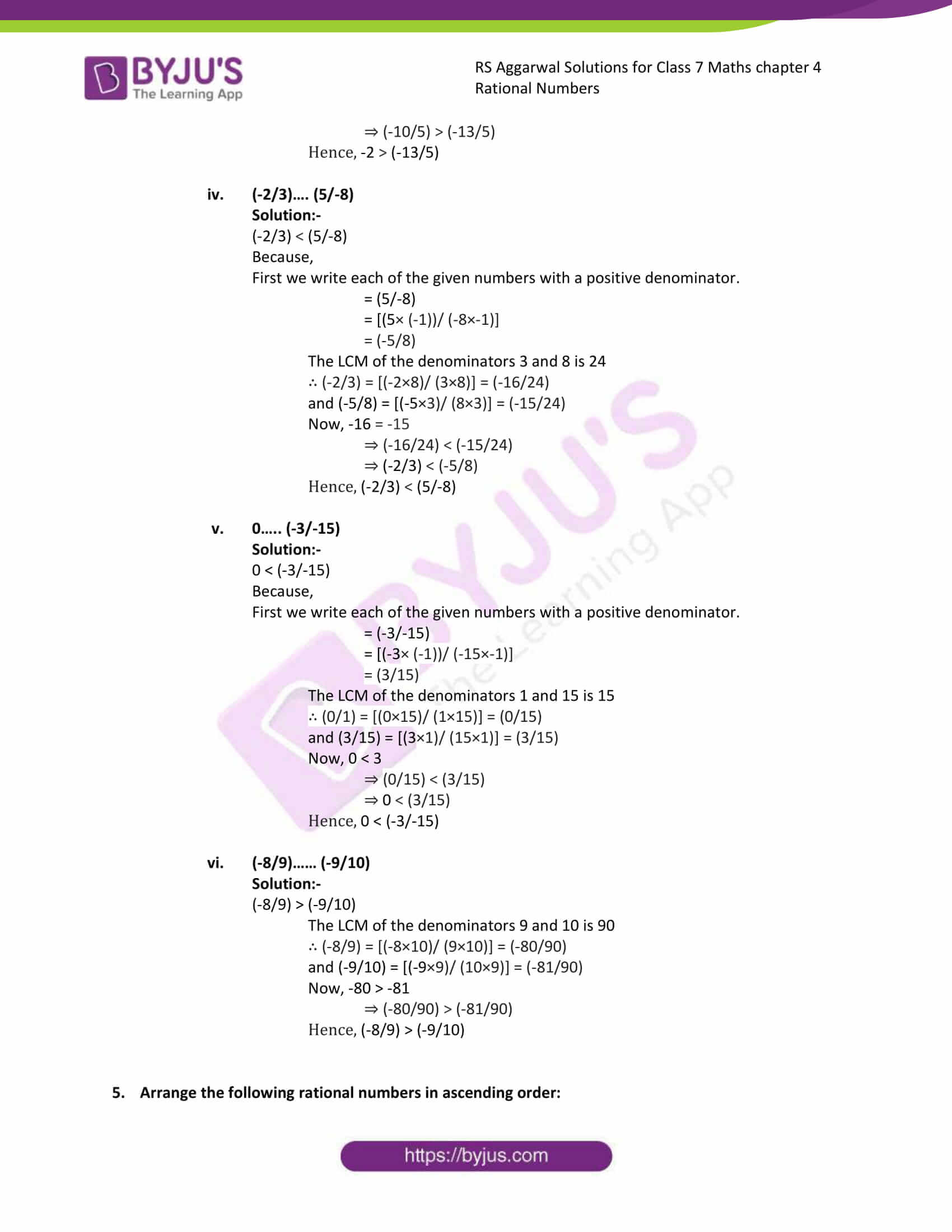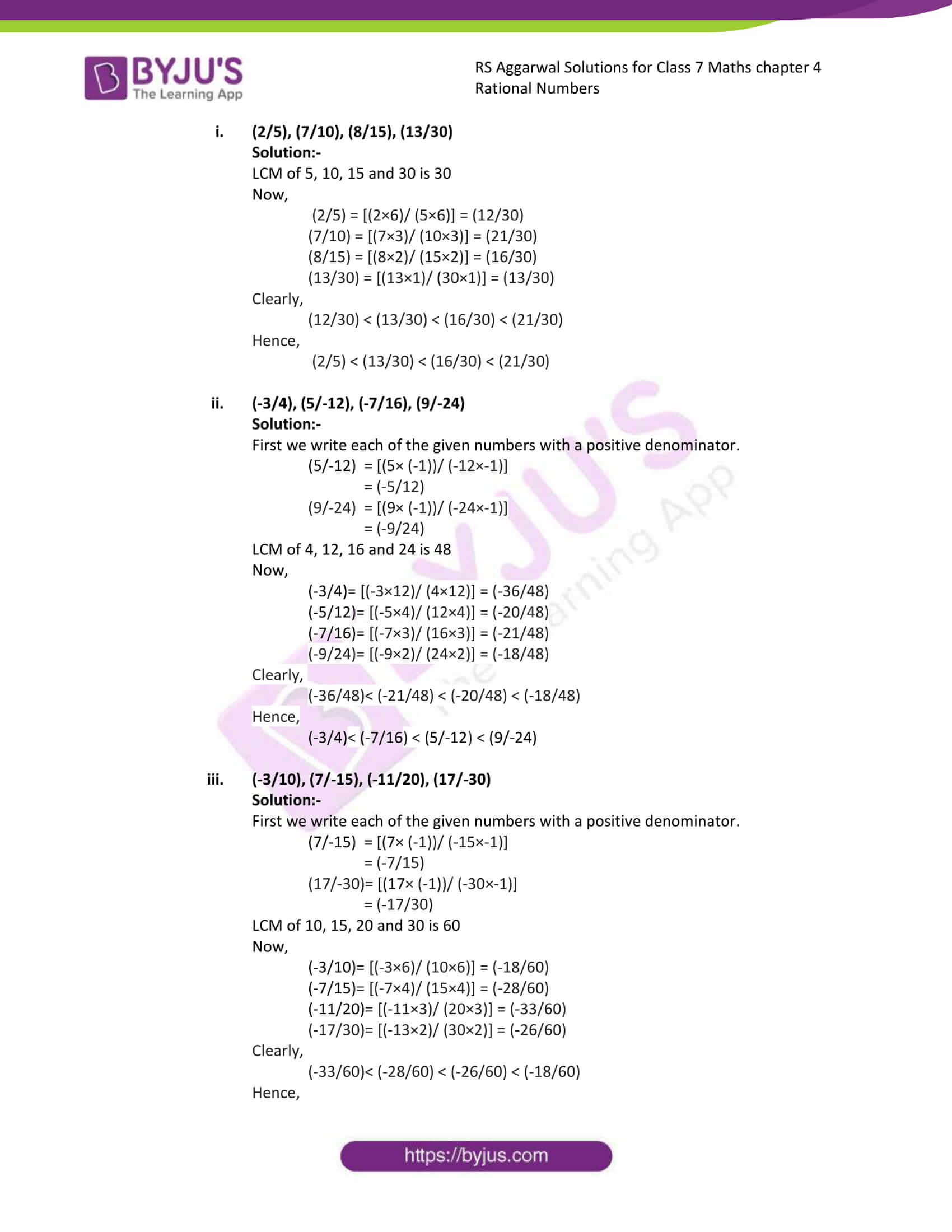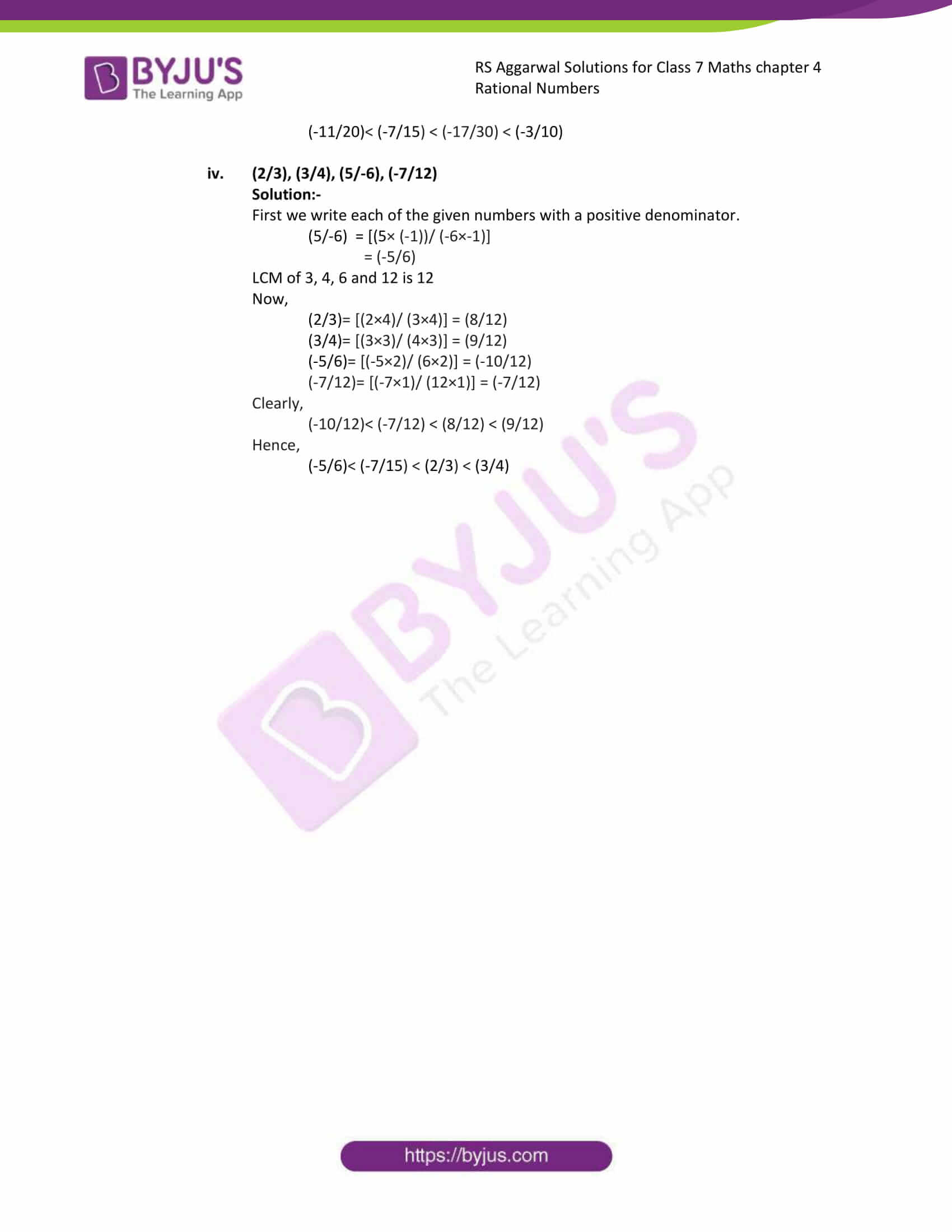### Access answers to Maths RS Aggarwal Solutions for Class 7 Chapter 4 – Rational Numbers Exercise 4B

1. Represent each of the following rational numbers on the number line: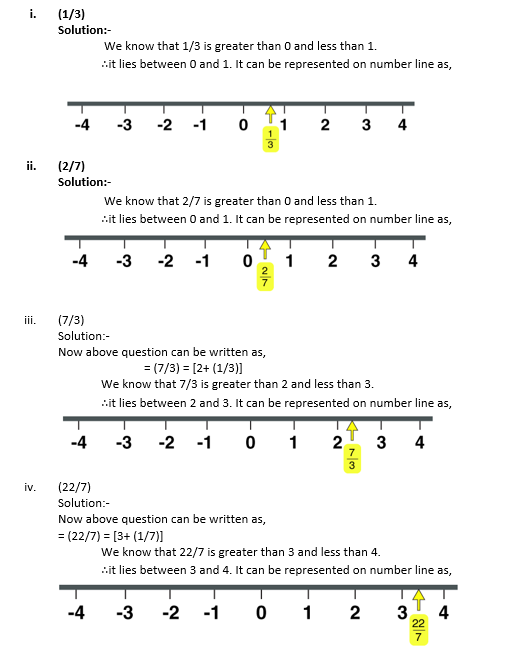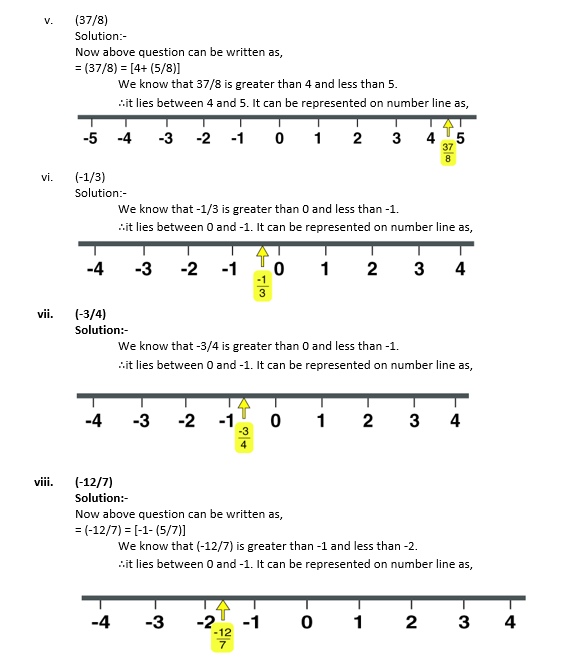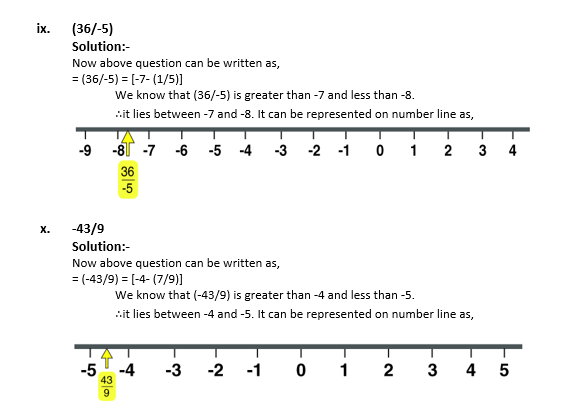2. Which of the two rational numbers is greater in each of the following pairs?
(i) (5/6) or 0

Solution:-

Since every positive rational number is greater than 0.

We have:

= (5/6) > (0)

(ii) (-3/5) or 0

Solution:-

Since every negative rational number is less than 0.

We have:

= (-3/5) < 0

(iii) (5/8) or (3/8)

Solution:-

Since both denominators are same therefore compare the numerators.

We have,

= 5 > 3

∴ (5/8) > (3/8)

(iv) (7/9) or (-5/9)

Solution:-

Since both denominators are same therefore compare the numerators.

We have,

= 7 > -5

∴ (7/9) > (-5/9)

(v) (-6/11) or (5/11)

Solution:-

Since both denominators are same therefore compare the numerators.

We have,

= -6 < 5

∴ (-6/11) < (5/11)

(vi) (-15/4) or (-17/4)

Solution:-

Since both denominators are same therefore compare the numerators.

We have,

= -15 > -17

∴ (-15/4) > (-17/4)

3. Which of the two rational number is grater in each of the following pairs?
(i) (5/9) or (-3/8)

Solution:-

The LCM of the denominators 9 and 8 is 72

∴ (5/9) = [(5×8)/ (9×8)] = (40/72)

and (-3/8) = [(-3×9)/ (8×9)] = (-27/72)

Now, 40 > -27

⇒ (40/72) > (-27/72)

Hence, (5/9) > (-3/8)

∴ 5/9 is greater.

(ii) (4/-3) or (-8/7)

Solution:-

First we write each of the given numbers with a positive denominator.

= (4/-3)

= [(4× (-1))/ (-3×-1)]

= (-4/3)

The LCM of the denominators 3 and 7 is 21

∴ (-4/3) = [(-4×7)/ (3×7)] = (-28/21)

and (-8/7) = [(-8×3)/ (7×3)] = (-24/21)

Now, -28 < -24

⇒ (-28/21) < (-24/21)

⇒ (-4/3) < (-8/7)

Hence, (4/-3) < (-8/7)

∴ -8/7 is greater.

(iii) (-12/5) or -3

Solution:-

The LCM of the denominators 5 and 1 is 5

∴ (-12/5) = [(-12×1)/ (5×1)] = (-12/5)

and (-3/1) = [(-3×5)/ (1×5)] = (-15/5)

Now, -12 > -15

⇒ (-12/5) > (-15/5)

Hence, (-12/5) > (-3)

∴ -3 is greater.

(iv) (7/-9) or (-5/8)

Solution:-

First we write each of the given numbers with a positive denominator.

= (7/-9)

= [(7× (-1))/ (-9×-1)]

= (-7/9)

The LCM of the denominators 9 and 8 is 72

∴ (-7/9) = [(-7×8)/ (9×8)] = (-56/72)

and (-5/8) = [(-5×9)/ (8×9)] = (-45/72)

Now, -56 < -45

⇒ (-56/72) < (-45/72)

⇒ (-7/9) < (-5/8)

Hence, (7/-9) < (-5/8)

∴ (-5/8) is greater.

(v) (4/-5) or (-7/8)

Solution:-

First we write each of the given numbers with a positive denominator.

= (4/-5)

= [(4× (-1))/ (-5×-1)]

= (-4/5)

The LCM of the denominators 5 and 8 is 40

∴ (-4/5) = [(-4×8)/ (5×8)] = (-32/40)

and (-7/8) = [(-7×5)/ (8×5)] = (-35/40)

Now, -32 > -35

⇒ (-32/40) > (-35/40)

⇒ (-4/5) > (-7/8)

Hence, (4/-5) > (-7/8)

∴ (4/-5) is greater.

(vi) (9/-13) or (7/-12)

Solution:-

First we write each of the given numbers with a positive denominator.

One number = (9/-13)

= [(9× (-1))/ (-13×-1)]

= (-9/13)

One number = (7/-12)

= [(7× (-1))/ (-12×-1)]

= (-7/12)

The LCM of the denominators 13 and 12 is 156

∴ (-9/13) = [(-9×12)/ (13×12)] = (-108/156)

and (-7/12) = [(-7×13)/ (12×13)] = (-91/156)

Now, -108 < -91

⇒ (-108/156) < (-91/156)

⇒ (-9/13) < (-7/12)

Hence, (9/-13) < (7/-12)

∴ (7/-12) is greater.

4. Fill in the blanks with the correct symbol out of >, < and =:
(i) (-3/7)….. (6/-13)

Solution:-

(-3/7) > (6/-13)

Because,

First we write each of the given numbers with a positive denominator.

= (6/-13)

= [(6× (-1))/ (-13×-1)]

= (-6/13)

The LCM of the denominators 7 and 13 is 91

∴ (-3/7) = [(-3×13)/ (7×13)] = (-39/91)

and (-6/13) = [(-6×7)/ (13×7)] = (-42/91)

Now, -39 > -42

⇒ (-39/91) > (-42/91)

⇒ (-3/7) > (-6/13)

Hence, (-3/7) > (6/-13)

(ii) (5/-13)…. (-35/91)

Solution:-

(5/-13)= (-35/91)

Because,

First we write each of the given numbers with a positive denominator.

= (5/-13)

= [(5× (-1))/ (-13×-1)]

= (-5/13)

The LCM of the denominators 13 and 91 is 91

∴ (-5/13) = [(-5×7)/ (13×7)] = (-35/91)

and (-35/91) = [(-35×1)/ (91×1)] = (-35/91)

Now, -35 = -35

⇒ (-35/91) = (-35/91)

⇒ (-5/13) = (-35/91)

Hence, (5/-13) = (-35/91)

(iii) -2…. (-13/5)

Solution:-

-2 > (-13/5)

Because,

The LCM of the denominators 1 and 5 is 5

∴ (-2/1) = [(-2×5)/ (1×5)] = (-10/5)

and (-13/5) = [(-13×1/ (5×1)] = (-13/5)

Now, -10 > -13

⇒ (-10/5) > (-13/5)

Hence, -2 > (-13/5)

(iv) (-2/3)…. (5/-8)

Solution:-

(-2/3) < (5/-8)

Because,

First we write each of the given numbers with a positive denominator.

= (5/-8)

= [(5× (-1))/ (-8×-1)]

= (-5/8)

The LCM of the denominators 3 and 8 is 24

∴ (-2/3) = [(-2×8)/ (3×8)] = (-16/24)

and (-5/8) = [(-5×3)/ (8×3)] = (-15/24)

Now, -16 = -15

⇒ (-16/24) < (-15/24)

⇒ (-2/3) < (-5/8)

Hence, (-2/3) < (5/-8)

(v) 0….. (-3/-15)

Solution:-

0 < (-3/-15)

Because,

First we write each of the given numbers with a positive denominator.

= (-3/-15)

= [(-3× (-1))/ (-15×-1)]

= (3/15)

The LCM of the denominators 1 and 15 is 15

∴ (0/1) = [(0×15)/ (1×15)] = (0/15)

and (3/15) = [(3×1)/ (15×1)] = (3/15)

Now, 0 < 3

⇒ (0/15) < (3/15)

⇒ 0 < (3/15)

Hence, 0 < (-3/-15)

(vi) (-8/9)…… (-9/10)

Solution:-

(-8/9) > (-9/10)

The LCM of the denominators 9 and 10 is 90

∴ (-8/9) = [(-8×10)/ (9×10)] = (-80/90)

and (-9/10) = [(-9×9)/ (10×9)] = (-81/90)

Now, -80 > -81

⇒ (-80/90) > (-81/90)

Hence, (-8/9) > (-9/10)

5. Arrange the following rational numbers in ascending order:
(i) (2/5), (7/10), (8/15), (13/30)

Solution:-

LCM of 5, 10, 15 and 30 is 30

Now,

(2/5) = [(2×6)/ (5×6)] = (12/30)

(7/10) = [(7×3)/ (10×3)] = (21/30)

(8/15) = [(8×2)/ (15×2)] = (16/30)

(13/30) = [(13×1)/ (30×1)] = (13/30)

Clearly,

(12/30) < (13/30) < (16/30) < (21/30)

Hence,

(2/5) < (13/30) < (16/30) < (21/30)

(ii) (-3/4), (5/-12), (-7/16), (9/-24)

Solution:-

First we write each of the given numbers with a positive denominator.

(5/-12) = [(5× (-1))/ (-12×-1)]

= (-5/12)

(9/-24) = [(9× (-1))/ (-24×-1)]

= (-9/24)

LCM of 4, 12, 16 and 24 is 48

Now,

(-3/4)= [(-3×12)/ (4×12)] = (-36/48)

(-5/12)= [(-5×4)/ (12×4)] = (-20/48)

(-7/16)= [(-7×3)/ (16×3)] = (-21/48)

(-9/24)= [(-9×2)/ (24×2)] = (-18/48)

Clearly,

(-36/48)< (-21/48) < (-20/48) < (-18/48)

Hence,

(-3/4)< (-7/16) < (5/-12) < (9/-24)

(iii) (-3/10), (7/-15), (-11/20), (17/-30)

Solution:-

First we write each of the given numbers with a positive denominator.

(7/-15) = [(7× (-1))/ (-15×-1)]

= (-7/15)

(17/-30)= [(17× (-1))/ (-30×-1)]

= (-17/30)

LCM of 10, 15, 20 and 30 is 60

Now,

(-3/10)= [(-3×6)/ (10×6)] = (-18/60)

(-7/15)= [(-7×4)/ (15×4)] = (-28/60)

(-11/20)= [(-11×3)/ (20×3)] = (-33/60)

(-17/30)= [(-13×2)/ (30×2)] = (-26/60)

Clearly,

(-33/60)< (-28/60) < (-26/60) < (-18/60)

Hence,

(-11/20)< (-7/15) < (-17/30) < (-3/10)

(iv) (2/3), (3/4), (5/-6), (-7/12)

Solution:-

First we write each of the given numbers with a positive denominator.

(5/-6) = [(5× (-1))/ (-6×-1)]

= (-5/6)

LCM of 3, 4, 6 and 12 is 12

Now,

(2/3)= [(2×4)/ (3×4)] = (8/12)

(3/4)= [(3×3)/ (4×3)] = (9/12)

(-5/6)= [(-5×2)/ (6×2)] = (-10/12)

(-7/12)= [(-7×1)/ (12×1)] = (-7/12)

Clearly,

(-10/12)< (-7/12) < (8/12) < (9/12)

Hence,

(-5/6)< (-7/15) < (2/3) < (3/4)

### Access other exercises of RS Aggarwal Solutions For Class 7 Chapter 4 – Rational Numbers

Exercise 4G Solutions